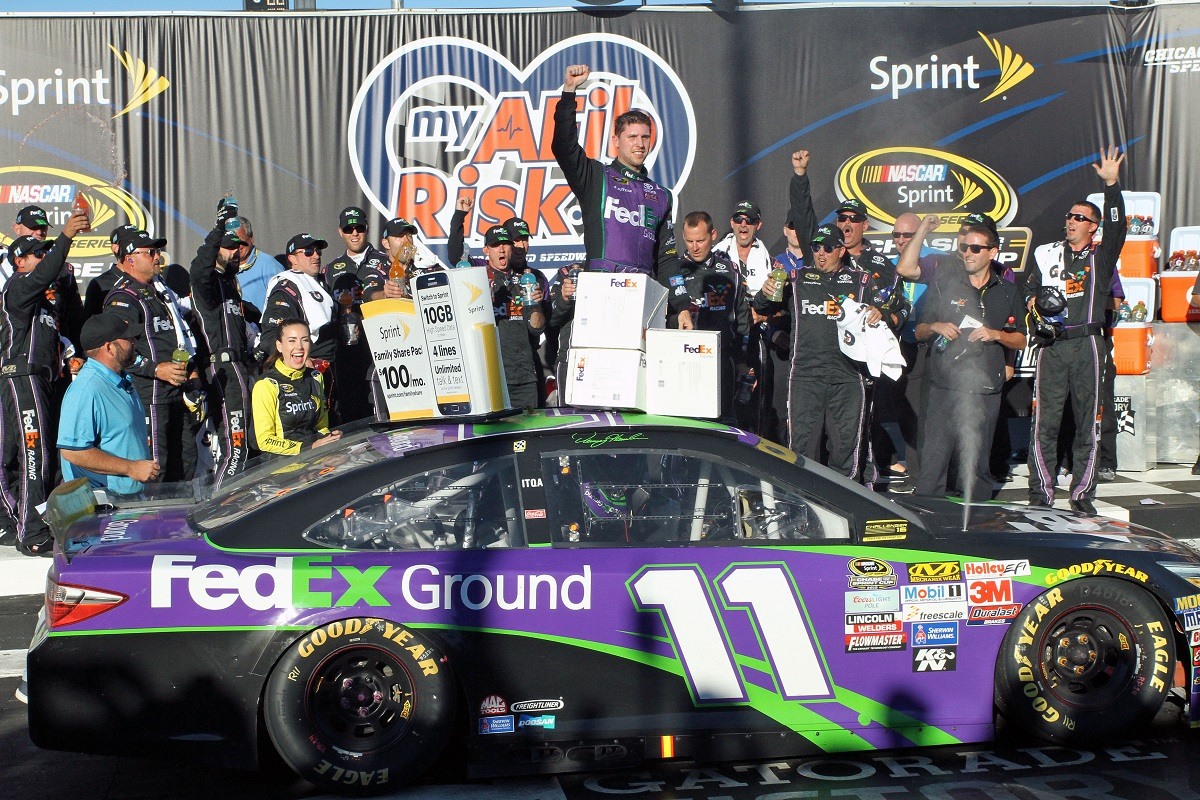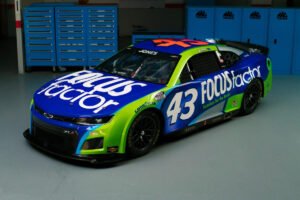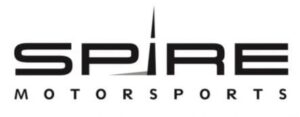December 1, 2021

# OnPitRoad.com Pick ‘Em Fantasy Pick Results for MYAFIBRISK.COM 400The Picks

Alex George, Brandon Feeney and Ronald Costigan picked Kevin Harvick, Jimmie Johnson and Dale Earnhardt Jr. Harvick finished 42nd with Johnson 11th and Earnhardt 12th. Points: 3+33+32=68

Ashley McCubbin picked Kevin Harvick, Kyle Busch and Jimmie Johnson. Busch finished ninth. Points: 3+37+33=73

Justin Tucker picked Brad Keselowski, Kevin Harvick and Joey Logano. Keselowski finished eighth with Logano sixth. Points: 36+3+39=78

Robert Hill picked Casey Mears, Ryan Blaney and David Ragan. Mears finished 20th with Blaney failed to qualify with Ragan finishing 15th. Points: 24+0+29=53

Brooke Clarke picked Kevin Harvick, Denny Hamlin and Jeff Gordon. Hamlin won with Gordon finishing 14th. Points: 3+47+31=81

Kreistina Blinova picked Kevin Harvick, Jimmie Johnson and Jeff Gordon. Points: 3+33+31=67

Marshall Gabell picked Matt Kenseth, Joey Logano and Jimmie Johnson. Kenseth finished fifth. Points: 40+39+33=112

Kyle Magda picked Sam Hornish Jr., Kyle Busch and A.J. Allmendinger. Hornish finished 30th with Allmendinger 36th. Points: 14+37+8=59

Steve Love picked Brad Keselowski, Matt Kenseth and Kurt Busch. Busch finished third. Points: 36+40+42=118

Tim Johnston picked Kevin Harvick, Joey Logano and Jimmie Johnson. Points: 3+39+33=75

Jeni Harris picked Carl Edwards, Matt Kenseth and Martin Truex Jr. Edwards finished second with Truex 13th. Points: 43+40+32=115

Doug Townsend picked Brad Keselowski, Kevin Harvick and Carl Edwards. Points: 36+3+43=82

Parker Ledger picked Kevin Harvick, Jeff Gordon and Dale Earnhardt Jr. Points: 3+31+32=66

Rory Townsend picked Kevin Harvick, Matt Kenseth and Ryan Newman. Newman finished fourth. Points: 3+40+40=83

Mike Cousins picked Brad Keselowski, Jeff Gordon and Jimmie Johnson. Point: 36+31+33=100

Jeffrey Breazeale picked Tony Stewart, Jeff Gordon and A.J. Allmendinger. Stewart finished 25th. Points: 19+31+8=58

Stephen Placido picked Joey Logano, Jimmie Johnson and Dale Earnhardt Jr. Points: 39+33+32=104

The Results/Fall Cup Standings

1. Steve Love – 118
2. Jeni Harris – 115
3. Marshall Gabell – 112
4. Stephen Placido – 104
5. Mike Cousins – 100
6. Rory Townsend – 83
7. Doug Townsend – 82
8. Brooke Clarke – 81
9. Justin Tucker – 78
10. Tim Johnston – 75
11. Ashley McCubbin – 73
12. Alex George, Brandon Feeney and Ronald Costigan – 68
13. Kreistina Blinova – 67
14. Parker Ledger – 66
15. Kyle Magda – 59
16. Jeffrey Breazeale – 58
17. Robert Hill – 53

Full Season Sprint Cup Series Standings

1. Stephen Placido – 2653+104=2757
2. (Points Leader Post Kentucky) Alex George – 2679+68=2747 (-10)
3. Justin Tucker – 2661+78=2739 (-18)
4. Steve Love – 2593+118=2711 (-38)
5. Tim Johnston – 2614+75=2689 (-60)
6. Parker Ledger – 2620+66=2686 (-63)
7. Doug Townsend – 2586+82=2668 (-81)
8. Ronald Costigan – 2583+68=2651
9. Ashley McCubbin – 2556+73=2629
10. Kreistina Blinova – 2553+67=2620
11. Brandon Feeney – 2547+68=2615
12. Marshall Gabell – 2498+112=2610
13. Brooke Clarke – 2488+81=2569
14. Rory Townsend – 2486+83=2569
15. Mike Cousins – 2466+100=2566
16. Kyle Magda – 2432+59=2491
17. Jeffrey Breazeale – 2292+58=2350
18. Jeni Harris – 2213+115=2328
19. Robert Hill – 2087+53=2140

Fall Overall Standings

1. (Points Leader Post XFINITY Chicago) Steve Love – 66+130+118=314
2. Jeni Harris – 85+107+115=307 (-7)
3. Mike Cousins  – 74+108+100=282 (-32)
4. Rory Townsend – 83+94+83=260 (-54)
5. Justin Tucker – 76+100+78=254 (-60)
6. Marshall Gabell – 69+71+112=252 (-62)
7. Brandon Feeney – 74+109+68=251 (-63)
8. Stephen Placido – 61+83+104=249 (-65)
9. Doug Townsend – 72+94+82=248 (-66)
10. Ronald Costigan – 68+110+68=244 (-70)
11. Parker Ledger – 79+97+66=242 (-72)
12. Robert Hill – 77+111+53=241 (-73)
13. Kreistina Blinova – 61+111+67=239 (-75)
14. Jeffrey Breazeale – 81+92+58=231 (-83)
15. Ashley McCubbin – 68+87+73=227 (-87)
16. Tim Johnston – 80+71+75=226 (-88)
17. Brooke Clarke – 61+71+81=213
18. Alex George – 58+84+68=210
19. Kyle Magda – 69+62+59=190

Full Season Overall Points Standings

1. (Points Leader Post Cup Kansas) Justin Tucker – 6766+76+100+78=7020
2. Ronald Costigan – 6629+68+110+68=6875 (-145)
3. Ashley McCubbin – 6582+68+78+73=6801
4. Brandon Feeney – 6483+74+109+68=6734
5. Stephen Placido – 6432+61+83+104=6680
6. Doug Townsend – 6410+72+94+82=6658
7. Steve Love – 6336+66+130+118=6650
8. Parker Ledger – 6287+79+97+66=6529
9. Mike Cousins – 6121+74+108+100=6503
10. Kyle Magda – 6304+69+62+59=6494
11. Jeffrey Breazeale – 6252+81+92+58=6483
12. Brooke Clarke – 6261+61+71+81=6474
13. Tim Johnston – 6243+80+71+75=6469
14. Kreistina Blinova – 6203+61+111+67=6442
15. Marshall Gabell – 6135+69+71+112=6387
16. Alex George – 6100+58+84+68=6310
17. Jeni Harris – 5951+85+107+115=6258
18. Rory Townend – 5847+83+94+83=6107
19. Robert Hill – 5522+77+111+53=5763

#### You may have missed#### Why Progressive Insurance is the right choice#### FOCUSFACTOR® TO PARTNER WITH RICHARD PETTY MOTORSPORTS#### Spire Motorsports to Field NASCAR Camping World Truck Series Team in 2022